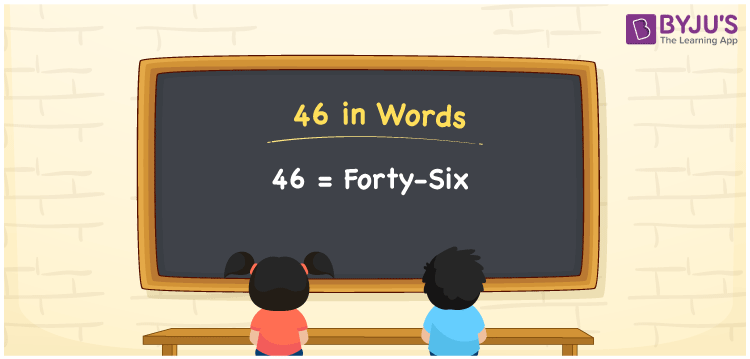# 46 in words

46 in words can be written as Forty-six. In this article, you will learn briefly about how to write the number 46 in words. For example, if you buy 5 eggs for Rs. 46 at the provision store, then you can say that “I bought 5 eggs for Forty-six Rupees at the provision store”. For more ideas about converting numbers in words, make use of the study materials given at BYJU’S. So, the number 46 can be read as “Forty-six” in words.

 46 in words Forty-six Forty-six in Numbers 46

## 46 in English Words## How to Write 46 in Words?

Learn in detail about the place value chart for 46 in words using the table. There are two digits in 46. Given below is the place value chart which shows the place value of the number 46 in a brief way.

 Tens Ones 4 6

The expanded form of 46 is:

4 × Ten + 6 × One

= 4 × 10 + 6 × 1

= 40 + 6

= 46

= Forty-six

Therefore, 46 in words is written as Forty-six.

46 is a natural number that precedes 47 and succeeds 45.

46 in words – Forty-six

Is 46 an odd number? – No

Is 46 an even number? – Yes

Is 46 a perfect square number? – No

Is 46 a perfect cube number? – No

Is 46 a prime number? – No

Is 46 a composite number? – Yes

## Frequently Asked Questions on 46 in Words

Q1

### Write 46 in words.

46 can be written as “Forty-six” in words.
Q2

### What is the value of 50 minus 4?

The value of 50 minus 4 is 46.
Q3

### Is 46 an even number?

Yes, 46 is an even number as it is completely divisible by 2.